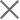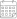Subscribe

### Indexes

June 2018 GDP Forecast | Despite optimistic growth forecasts, a growing trade deficit in April gives reasons to worry26 June 2018

ISET-PI has updated its forecast of Georgia’s real GDP growth rate for the second and third quarters of 2018. Here are the highlights of this month’s release:

Geostat has released its GDP growth estimate for the first quarter of 2018. The Q1 growth stands at 5.2%, which is 1.1 percentage points above the recent forecast.

ISET-PI’s forecast of real GDP growth for the second quarter of 2018 remains unchanged at 5.9%. The first estimate for the third-quarter growth forecast is at 7.2%.

Based on April’s data, we expect annual growth in 2018 to be 6.2% in the worst-case or “no growth” scenario, and 6.5% in the best-case or “average long-term growth” scenario. Our “middle-of-the-road” scenario (based on average growth over the last four quarters) predicts 6.3% real GDP growth in 2018.

Geostat has released its preliminary estimate of GDP growth for the first quarter of 2018. Its estimated growth figure is 5.9%, which is 1.1 percentage points higher than ISET PI’s forecast. As a result, our projected real GDP growth for the second quarter of 2018 has also been revised upward to 5.9%. Moreover, ISET PI’s first forecast of the third-quarter growth is also very optimistic, at 7.2%. These high forecast figures can be explained by the fact that quarterly growth rates typically follow a certain pattern, captured by ISET-PI’s empirical forecast model. Thus, the higher-than-usual growth in the first quarter may result in an overly optimistic forecast about the next quarter’s performance. Indeed, ISET PI’s forecasts for Q2 and Q3 are largely influenced by the high Q1 actual growth.